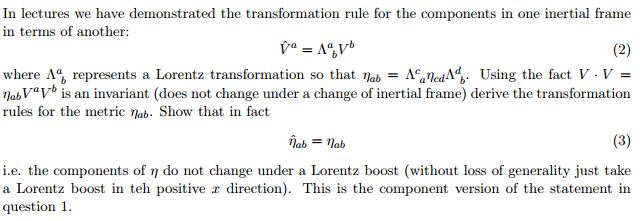Home / Answered Questions / Other / 2-in-lectures-we-have-demonstrated-the-transformation-rule-for-the-components-in-one-inertial-frame--aw554

# (Solved): (2) In Lectures We Have Demonstrated The Transformation Rule For The Components In One Inertial Fram...(2) In lectures we have demonstrated the transformation rule for the components in one inertial frame in terms of another: W = AV where A, represents a Lorentz transformation so that Nab = And Ad. Using the fact V.V = nab VV is an invariant (does not change under a change of inertial frame) derive the transformation rules for the metric Nab. Show that in fact lab = lab (3) i.e. the components of n do not change under a Lorentz boost (without loss of generality just take a Lorentz boost in teh positive x direction). This is the component version of the statement in question 1.

We have an Answer from Expert